Web www.handwritingforkids.com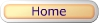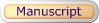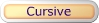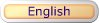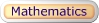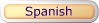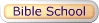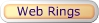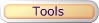### Mathematics - Subtraction

• Online / Interactive
• Printable w/guideline
• Printable
• Customized

This online/interactive math subtraction generator will provides plenty of online math practice, boost test scores and prepare the child/children to meet the Kindergarten, Grade 1, and Grade 2 curriculum standards. This online/interactive math subtraction, will create a vertical 1 digit by 1 digit (1x1) and 2 digits by 1 digit (2x1) equations with an option of timed test, and it will automatically graded/scored your results in a printable format.

Type of online math problems:

• Online Math 1 - Subtraction - Difference 0 to 5
• Online Math 1 - Subtraction - Difference 5 to 10
• Online Math 1 - Subtraction - Difference 0 to 10
• Online Math 1 - Subtraction - Difference 0 to 18
• Online Math 1 - Subtraction - Difference 10 to 18
 6 - 5 1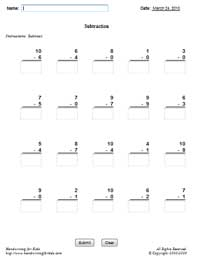This printable basic math subtraction worksheet generator will provides plenty of math practice, boost test scores and prepare the child/children to meet the Kindergarten, Grade 1, and Grade 2 curriculum standards. This math worksheet maker will create a vertical math subtraction, 1 digit by 1 digit (1x1) and 2 digits by 1 digit (2x1) equations with handwriting lines.

See sample math worksheets below:

 6 - 5 1

This printable basic math subtraction worksheet generator will provides plenty of math practice, boost test scores and prepare the child/children to meet the Kindergarten, Grade 1, and Grade 2 curriculum standards. This math worksheet maker will create a vertical math subtraction, 1 digit by 1 digit (1x1) and 2 digits by 1 digit (2x1) equations without handwriting lines.

See sample math worksheets below:

 6 - 5 1

This worksheet generator will create your own customized/personalized subtraction worksheet. You enter your own math problems in a printable worksheet. Maximum 5 digits x 5 digits (5x5).

 6 - 5 1• Online / Interactive
• Printable w/guideline
• Printable
• Customized

This online/interactive subtraction math drill maker will create 1 digit by 1 digit (1x1) and 2 digits by 1 digit (2x1) equations with an option of timed test, and it will automatically graded/scored your results in a printable format. Suggestive Grade Levels: Kindergarten, Grade 1, and Grade 2.

 12 - 4 = 8

 Type of online math problems: Online Math 2 - Subtraction - Difference 0 to 5 Online Math 2 - Subtraction - Difference 5 to 10 Online Math 2 - Subtraction - Difference 0 to 10 Online Math 2 - Subtraction - Difference 0 to 18 Online Math 2 - Subtraction - Difference 10 to 18

This printable basic math subtraction worksheet generator will provides plenty of math practice, boost test scores and prepare the child/children to meet the Kindergarten, Grade 1, and Grade 2 curriculum standards. This math worksheet maker will create a horizontal math subtraction, 1 digit by 1 digit (1x1) and 2 digits by 1 digit (2x1) equations with handwriting lines.

 12 - 4 = 8

 See sample math worksheets below:

This printable basic math subtraction worksheet generator will provides plenty of math practice, boost test scores and prepare the child/children to meet the Kindergarten, Grade 1, and Grade 2 curriculum standards. This math worksheet maker will create a horizontal math subtraction, 1 digit by 1 digit (1x1) and 2 digits by 1 digit (2x1) equations without handwriting lines.

 12 - 4 = 8

 See sample math worksheets below:

This worksheet generator will create your own customized/personalized subtraction worksheet. You enter your own vertical math problems in a printable worksheet. Maximum 5 digits x 5 digits (5x5).

 12 - 4 = 8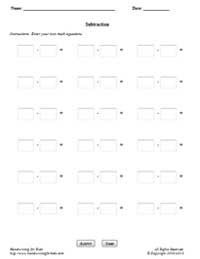• Online / Interactive
• Printable w/guideline
• Printable
• Customized

This online/interactive subtraction math drill maker will create 2 digits by 1 digit (2x1) equations with an options of "No Borrowing", "Borrowing", or "Mixed" (both borrowing/no borrowing) math equations, and timed test. Also, it will automatically graded/scored your results in a printable format. Note: This program will randomly select minuend numbers between 10-99, and subtrahend numbers between 0-9. Suggestive Grade Levels: Grade 1, Grade 2, and Grade 3.

See sample math worksheets below:

• Subtraction - No Borrowing
• Subtraction - Borrowing
• Subtraction - Mixed - Borrowing/No Borrowing
 46 - 4 42This printable math worksheet maker will create a vertical math subtraction, 2 digits by 1 digit (2x1) equations with an option of "No Borrowing", "Borrowing", or "Mixed" (both borrowing and no borrowing) math equations, using the handwriting lines.. Note: This program will randomly select minuend numbers between 10-99, and subtrahend numbers between 0-9.

See sample math worksheets below:

 46 - 4 42

This printable math worksheet maker will create a vertical math subtraction, 2 digits by 1 digit (2x1) equations with an option of "No Borrowing", "Borrowing", or "Mixed" (both borrowing and no borrowing) math equations, and without the handwriting lines.. Note: This program will randomly select minuend numbers between 10-99, and subtrahend numbers between 0-9.

See sample math worksheets below:

 46 - 4 42

This worksheet generator will create your own customized/personalized subtraction worksheet. You enter your own math problems in a printable worksheet. Maximum 5 digits x 5 digits (5x5).

 46 - 4 42### Subtraction - TablesExamples Subtraction Tables How to use the subtraction table Blank Table #1 Answer SheetSubtraction 0-12 Flash Cards (MS Word Document - Printable Only) Flash Cards Printing Instructions: 1. Print all odd pages 2. Flip the stack of printed pages and re-insert into the printer. 3. Print all even pages. Suggestion: Print on color paper or card-stock paper.

### JavaScript Interactive Math* - Simple Subtraction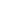Level 1: Visual Math (1 digit - between 0-9) Option 1: Graphical Input and AnswersExercise 1: Simple Additions with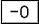Exercise 6: Simple Additions with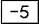Exercise 2: Simple Additions with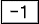Exercise 7: Simple Additions with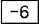Exercise 3: Simple Additions withExercise 8: Simple Additions with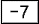Exercise 4: Simple Additions with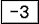Exercise 9: Simple Additions withExercise 5: Simple Additions withExercise 10: Simple Additions withOption 2: Graphical Input OnlyExercise 1: Simple Additions withExercise 6: Simple Additions withExercise 2: Simple Additions withExercise 7: Simple Additions withExercise 3: Simple Additions withExercise 8: Simple Additions withExercise 4: Simple Additions withExercise 9: Simple Additions withExercise 5: Simple Additions withExercise 10: Simple Additions withJavaScript Math (Interactive)*

* JavaScript must be enabled on your browser.

Sudoku for Kids (Picture Sudoku) 4x4